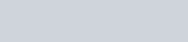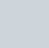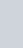Two coins are tossed simultaneously.
Question:

Two coins are tossed simultaneously. Find the probability of getting exactly one head.

Solution:

GIVEN: Two coins are tossed simultaneously.

TO FIND: Probability of getting exactly one head.

When two coins are tossed then the outcome will be

TT, HT, TH, HH.

Hence total number of outcome is 4.

Exactly one head we get 2 times

Hence total number of favorable outcome i.e. exactly one head is 2

We know that PROBABILITY =Hence probability of getting exactly one head =Hence probability of getting exactly one head =